## Saturday, April 30, 2011

### Manifolds: The Shape of the Universe II

This post is the penultimate segment of the Manifolds Series, and the second part concerning the Shape of the Universe. For the first, see here. For the first post of the entire series, see here.

The 3-torus theory of the Universe is relatively simple and elegant, but it is not the only candidate for the shape. The 3-torus represented finite Euclidean geometry in the debate for the Universe's global topology. This is because the eight corners of the cube eventually coincide when the faces are connected. It is clear that laying out eight corners "fills up" Euclidean 3-space. To see this, consider the 3-dimensional linear coordinate system.It has three axes, and splits space up into eight sections. At the origin, (the point of coincidence) the corner of each region is the corner of a cube. For more information about 3-dimensional angles (known as solid angles) see the beginning of Polytopes: Part III.

Of course, it is always possible that the Universe is simply infinite, and that it has no notable global topology. However, it is more logical, since the Universe was very probably at a finite size at some point in time, that it remains of measurable size. However, the curvature is not known for sure, and representatives for finite elliptic and hyperbolic geometry exist as well.

If the Universe is elliptic (a perspective which would have the Universe reversing in its expansion at some time in the future) it may be in the form of a 3-sphere, the simplest of elliptic 3-manifolds. Extending off of the common 2-sphere in three dimensions, the 3-sphere is the set of points in Euclidean four-dimensional space that are equidistant from a given fixed point. Its construction can be visualized as follows.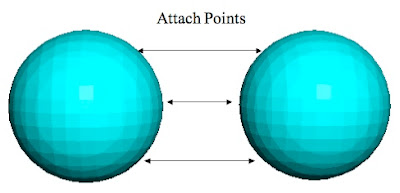It was discussed perviously that attaching the boundary of one disc to another results in the 2-sphere. Going up a dimension, the same goes for the 3-sphere. Two balls (solid spheres) have their boundaries attached in a one-to-one correspondence (as indicated by the arrows) and the resulting manifold is a 3-sphere, although the process itself cannot be visualized in 3-dimensional Euclidean space.

To imagine traveling through this space, visualize each ball as a set of concentric spheres. Starting at the center of the left ball, one would walk outward until reaching the boundary of the left ball, which, after the 3-sphere is constructed, is the same as the boundary of the right ball. One would then continue to walk in the same direction, reaching the center of the right ball. After that, the process would then reverse, and one would cross the boundary again, this time back into the left ball. It follows from the above construction that the centers of each ball become a pair of poles on the 3-sphere, diametrically opposite from each other.

Using the 2-sphere as an analog to how gravity works in this Universe, one can easily see that gravitational waves travel as arcs of great circles of the sphere. Unlike the torus, only two arcs (the major and minor arcs of a given great circle) connect two points, with an exception if the points are polar opposites, when an infinite number of gravitational rays connect two points. Therefore, the opposite pole is the "hot spot" for this manifold, where the net gravitational force is zero. In addition, due to the presence of the major arc component, the amount of gravity between two points in one direction is less then it "should" be, as the major arc component is subtracted (being in the opposite direction). These results are summarized in the figure below.In the above figure, the blue object attracts the green object (which has negligible mass) with a force equal to the minor arc gravitational pull minus the major arc gravitational pull in the opposite direction. These gravity vectors emanating from the blue object are the only two that intersect the green object, if both objects are treated as points. Again, this is similar to the sphere, where all pairs of points with the exception of anti-polar pairs have exactly two geodesics connecting them.

Finally, it is possible that Universe is hyperbolic. The leading theory for a hyperbolic Universe is known as the Picard horn. The two-dimensional analog for this manifold is the pseudosphere:This 2-manifold is infinite in extent, but, remarkably, has finite surface area and finite volume. As an interesting addendum, the surface area of the psuedosphere is equal to that of a sphere of the same radius. The geodesics on this manifold are called tractrices, circles, and rotating tractrices, all of which are illustrated below (click to enlarge).The view above is actually of the half-psuedosphere, and it is often used to represent a two-dimensional hyperbolic plane. A point on this manifold can be identified by its height off the base, and the angle around the central axis. The geodesic of constant height is the circle, the geodesic of constant angle is the tractrix, and every other geodesic has a change in height proportional to a change in angle, in other words, a linear function of the angle dependent on the height. This general geodesic is a rotating tractrix, and can (as shown above) travel around the entire pseudosphere any number of times.

If two points do not lie at the same height on the pseudosphere, then there are an infinite number of rotating tractrices connecting them. Again taking these to be gravitational waves, the "hot spots" of net zero force are the points 180º separated (on opposite sides) but at the same height. If two points are 180º separated but are not at the same height, then the net gravitational force would be to decrease their separation in height. These and other properties are summarized below.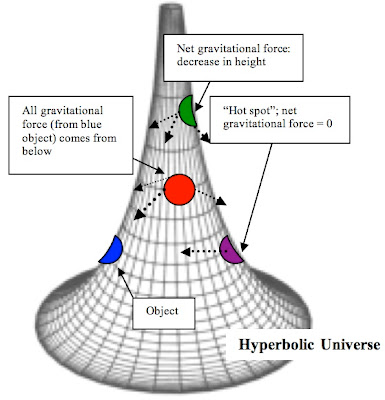The properties of the pseudosphere Universe are similar to that of the torus Universe, with the excpetion that there is only one class of non-contractible loops on the surface, (cricles) wheareas a torus has two: one going around the ring, and the other around the hole in the center. Therefore, as shown above, gravitational rays from the blue object to a higher one, namely the red, can only approach it from below, as opposed to the torus, where gravitational rays could approach from all directions.

The true hyperbolic plane is in some ways different from the psuedosphere, but it serves well as an example, and the three dimensional equivalent is notable for having finite volume, and a Universe of this type would also be finite, despite (again) being infinite in extent.

The above three possibilities are among the most prominent theories for the shape of the Universe. But which of these reflects the current visual evidence? This is the topic of the final post of the Manifolds Series.

Sources: http://en.wikipedia.org/wiki/Shape_of_the_Universe, The Poincare Conjecture by Donal O'Shea, http://www.ams.org/notices/200406/fea-weeks.pdf

## Friday, April 22, 2011

### Manifolds: The Shape of the Universe

This post is part of The Manifolds Series.

By use of maps, the surface of the Earth can be definitively defined as a sphere. However, if one only specifies that going in any direction on the Earth will eventually return one to his or her starting point, then many different manifolds qualify. The Earth could have just as easily been a torus, or any other finite manifold without an edge. Only by confirming the curvature within several different "patches" of a manifold can one uniquely determine it.

The same problem exists with the Universe. However, it is a rather more difficult one.

A common misconception concerning the shape of the Universe is that if it is finite, it has an edge or boundary. This is not true. On a 3-sphere, (recall that the surface of the Earth is a 2-sphere) one could go indefinitely through 3-dimensional space in one direction and never reach an edge, although he or she may return to his or her starting point. In fact, the Universe's lack of an edge is mostly agreed upon, as there would be disagreements in density and isotropy that make an edge unlikely.

Also, the curvature of the Universe can be determined to a fairly accurate degree by measuring its density. The density of matter in the Universe determines how fast it continues to expand. This is because the amount of gravity counteracting the expansion of the Universe is dependent on the amount of matter and energy present, and this in turn, determines whether the Universe is expanding, and at what rate. Since the presence of matter also determines how space is bent, the local curvature of the Universe around the aforementioned matter can be calculated as spherical, Euclidean, or hyperbolic.

Throughout all of the Universe that we can see (the observable Universe) the matter seems evenly distributed at a sufficiently high scale. Obviously, on (relatively) small scales, there is a large difference in density between stars and the interstellar medium, and between galaxies and the intergalactic void, but when one considers density on the scale of billions of light-years, the density is remarkably uniform. From this, one can assume that the curvature of the Universe is constant.

Additionally, observations up to the present have suggested that the density of the Universe is very close to the so called "critical density", at which the curvature of the Universe would be exactly 0. The constant Ω, known as the density parameter, representing the ratio of the actual density to the critical density, would then be 1 for a flat Universe, greater than 1 for a spherical Universe, and less than 1 for a hyperbolic one.

As a caveat to assumptions about the finite or infinite nature of the Universe, note that the curvature of the Universe does not determine its global structure topologically. Many people assume that if the Universe has flat geometry, it must be infinite, just as the flat Euclidean plane is. However, it was noted in previous posts that the torus has Euclidean geometry as well.

In fact, when one considers 3-manifolds, there are 10 possibilities for finite Euclidean 3-manifolds alone, the most simple of which is the 3-torus.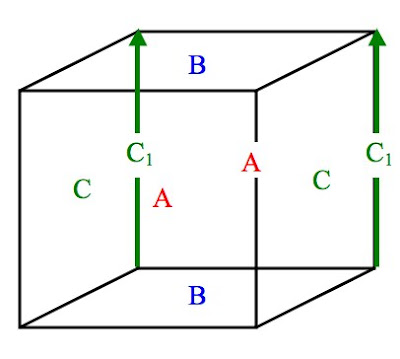The fundamental cube for the 3-torus. Imagine that the upper right end face of the cube is the front. Each face is connected with the opposite one (front to back, left to right, top to bottom) while preserving the orientation of faces. This means that the edge C1 is attached (facing up) to the corresponding edge also labeled C1 on the opposite C face.

Many telltale signs would exist if the Universe was a 3-torus. Using the 2-torus (previously known as simply the torus) as an analog, one can explore the remarkable phenomena of a toroidal Universe. To do this, consider life forms occupying the surface of a 2-torus, and then consider the 3-dimensional extension.

The first of these is the closed and finite nature of this Universe. An inhabitant of the 2-torus would see another copy of himself looking around the top of the donut! (see below)Inhabitants of this Universe would see an image of themselves by looking in the direction indicated by the arrow. The light itself actually leaves the other side of the blue oval, and travels along the orange path, reaching the blue oval again after one revolution. One might argue that the curvature of the torus would obscure the view, but this is not true, as the Universe is the surface of the torus, and light can only travel along this surface. Therefore, light would traverse a closed circle in certain directions. In fact, the number of these directions is infinite!

By looking around the "ring" of the torus, inhabitants of the blue oval might also see themselves. The same goes for an observer looking diagonally, where the light would circle around the bottom as it goes around, any number of times! Infinite images of the same blue oval would exist in their "sky"!

Extending this system to our Universe, the 3-torus shape could easily be identified by images of our galaxy, the Milky Way, in the night sky, right? Unfortunately, it isn't that easy. First, the sheer size of the Universe may be so large that even the closest image point may be many billions of light years away-beyond the scope of the observable Universe. And even if there was an image point within our view, we couldn't easily identify it, as it would be an image of the galaxy from billions of years ago! Although we may not know it, a galaxy looked upon by the Hubble could possibly be our own, simply at a different stage of evolution!

How then, does a donut Universe leave its mark? The signs may be more subtle, but they are there. For example, consider a point in space emitting light uniformly in all directions. Assuming that the intensity of the light dies away over distance, one would expect that the brightness at a constant distance would remain constant. This is not the case.

Returning to our above example, light rays would converge on the opposite side of the torus, and in multiple places in between. By observing from each and every point on the torus, one could construct a contour diagram of the brightness compared to what would otherwise be expected. Judging from their distance from the source, some points receive more light than they "should" in a generic flat, infinite Universe. The two points that deviate most are the diametrically opposite point on the torus, and the point on the bottom of the ring. Other points would be intermediately shaded. These "hot spots" are indicative of a donut Universe, but there are too many light sources for the specific example above to take effect.

Finally, perhaps the most important phenomenon is the discrepancies in gravity that would occur. Treating gravity waves similar to the light example above, one can clearly see that an object, which exerts gravity on its surroundings uniformly, would exert more gravity on some point in the toroidal Universe than others. The only difference here is that direction matters. For an object on the exact opposite side of the torus Universe, the gravity waves converge from all directions in a symmetrical way, adding up to a net zero force. For an object in the vicinity of the original object, the convergence of gravitational waves adds more attraction to the object than one would normally expect given the distance for an infinite Universe. These results are summarized in the figure below with the two-dimensional analogs of both the flat and the toroidal Universe. (click to enlarge)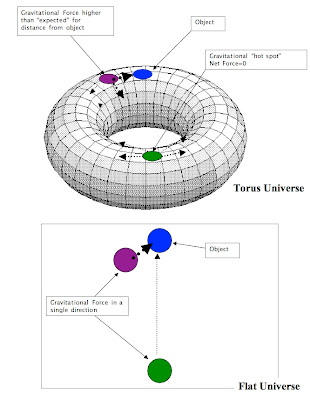In both figures, the dotted lines represent gravitational attraction. It is assumed for simplicity that the blue objects are the only bodies exerting gravity, and that the remainder of the objects are of negligible mass. In the flat Universe, only one line connects two points, but on the torus, multiple lines between two points are a result of the finite cyclic nature of the manifold; a line going around the manifold will come back to the vicinity of its original position.

In the next post of the Manifolds Series, other theories are considered.

Sources: http://en.wikipedia.org/wiki/Shape_of_the_Universe, The Road to Reality by Roger Penrose, The Poincare Conjecture by Donal O'Shea, http://www.astronomy.ohio-state.edu/~ryden/ast162_9/notes40.html, http://www.math.brown.edu/~banchoff/STG/ma8/papers/leckstein/Cosmo/torus.html

## Thursday, April 14, 2011

### Manifolds: Curvature and Construction II

This post is part of the Manifolds series. For the previous post in the series, see Manifolds: Curvature and Construction.

In the previous post, fundamental polygons were introduced. A further exploration of these figures is important in constructing manifolds. However, we find that there are limits to the accuracy to which even two-dimensional manifolds can be represented in three-dimensional space. Take the torus for example. It was found to have a fundamental polygon ABA*B*. All four corners of the four-sided fundamental polygon eventually coincide when the torus is constructed. As a result, the angular measure around the resulting point on the manifold is exactly 360º, and the manifold is Euclidean at that point.

This result can be expanded by considering the symmetry of the torus. In fact, any point can be chosen as the coincidence of the four corners. This means that the geometry around every point on a torus is perfectly flat.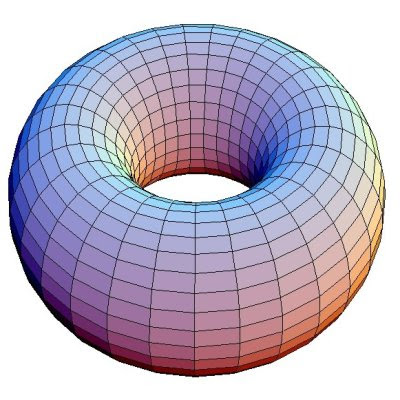However, an examination of the torus in three-space (above) suggests that this is not the case. What is happening here? The answer turns out to be that the folding of the cylinder to the torus distorts the final result, resulting in a manifold with areas of positive and negative curvature. The torus in its true form can only be expressed in four dimensions and higher, and is called a flat torus. Note that the manifold is not truly "flat", but can be constructed in four dimensions from a flat plane without distorting distances.

Similarly, the sphere (fundamental polygon ABB*A*) connects only two of opposite the corners together. The local geometry of a point is less than 360º, and the sphere therefore exhibits spherical geometry, an obvious fact.

The lapse in the accurate representation of 2-manifolds in 3-space is even more pronounced when one considers the fundamental polygon below.This fundamental polygon can also be written ABAB*. When constructed, this fundamental polygon takes the form of a manifold known as the Klein Bottle. The first step in construction is the same as the torus, i.e. connecting the sides marked B and forming a cylinder. However, the A sides are facing in opposite directions and the cylinder must be inverted through itself to construct the manifold. This construction is shown below.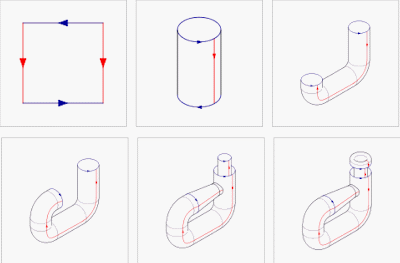The final product is the Klein Bottle.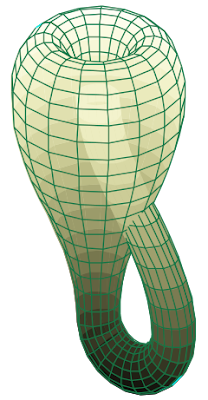It is apparent that self-intersection is necessary in order to construct this manifold in three dimensions. As a result, this too gives only a limited view of its actual structure. The addition of another dimension is needed to eliminate the self-intersection, and to show that this manifold too has Euclidean geometry everywhere.

Yet another fundamental polygon ABAB, produces another manifold that cannot be constructed in three dimensions without self-intersection. It is known as the real projective plane. It has many interesting properties, including its geometric construction. It is the most "difficult" to construct out of the fundamental squares because both pairs of opposite sides are orientated in different directions. Geometrically speaking, it is the set of all lines through the origin in Euclidean 3-space connected into a surface, although this gives little insight to its shape.

More complicated manifolds can be constructed from larger fundamental polygons (all with an even number of sides, of course, because one side must line up with another). For example, consider the hexagon ABCC*B*A* (shown below).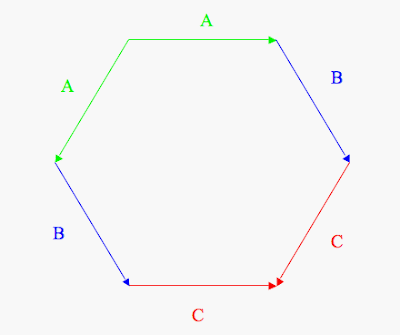This fundamental polygon produces the sphere, just as the ABB*A* does, since only a single diagonal fold is necessary to connect all of the indicated sides. Similarly, ABCB*A*C* produces the torus, ABCB*AC* the Klein Bottle, and ABCBAC, the real projective plane. These are all merely extensions of the fundamental squares, as two sides facing the same direction can be collapsed into one. Take for example the torus, ABCB*A*C*.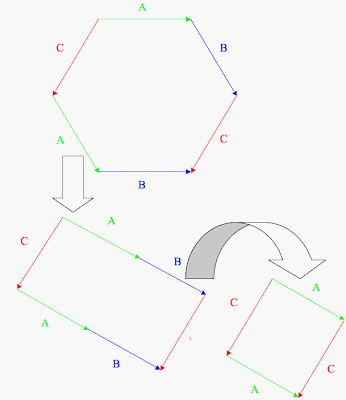Since continuous deformation still preserves the identity of a manifold, the same goes for its fundamental polygon. The above hexagon can therefore be adjusted into a rectangle. The sides A and B are in the same orientation and position relative to each other, they can be combined into a single side while still representing the same idea. The other examples above can be similarly collapsed into fundamental squares.

Fundamental polygon formulas also exist to generate the simplest surface of any given genus, i.e. the n-fold torus. For the two-holed torus, the fundamental polygon is the octagon ABA*B*CDC*D*, which under inspection, is simply two tori fundamental squares "added" together, corresponding to the "gluing" of two tori together to create the double torus. This is equal to ABA*B*+CDC*D*. All eight angles of the polygon coincide where the two tori share a boundary, and the double torus is therefore a hyperbolic manifold. (each angle of a octagon=135º, 8*135º=1080º>360º) With continuous deformation, the point of coincidence can be moved to any point on a double torus, and the manifold therefore has hyperbolic geometry anywhere.

As one adds more tori to the fundamental polygon, the manifold becomes increasingly distorted. It fact, it is "difficult" to draw an n-fold torus with more than three holes in Euclidean 3-space. The general formula is

ABA*B*A'B'A'*B'*A''B''A''*B''*...

which produces an n-fold torus, with each sequence of four letters (ABA*B*, A'B'A'*B'*, A''B''A''*B''*, etc.) represents a single torus that is glued to all others.

As with many aspects of manifolds, the idea of a fundamental polygon may be extended to higher dimensions. For 3-manifolds, fundamental polyhedra take on this role. For example, one might specify a manifold as the resulting figure when opposite faces of a cube are connected. Such connections cannot be visualized in Euclidean 3-space.

Finally, since deformation is permitted, even differently shaped n-faces of any closed polytope of any dimension can be connected to form a manifold. Untold multitudes of manifolds can be produced this way.

The next post of the Manifolds Series deals with the application of manifolds to the shape of the Universe.

Sources: http://en.wikipedia.org/wiki/Fundamental_polygon, The Poincare Conjecture by Donal O'Shea

## Wednesday, April 6, 2011

### Manifolds: Curvature and Construction

This is part of a series on Manifolds. For more information about subjects mentioned, see The Complete Manifolds Series.

On the previous post, it became possible to define unique points on a manifold by the use of coordinates. Consequently, the connecting of these points forms lines.

However, if one insists on staying on the surface of a manifold, the lines will not necessarily be straight. Take the sphere for example. Any lines drawn on the surface will inevitably be curved. But what does curvature mean? And how does one choose the single line that connects two points? The answer is a simple one.

On a surface, the line between two points is the shortest curve that connects them. On a plane, these lines are straight. On a sphere, the lines are arcs, part of great circles whose radius is that of the sphere itself (see below). On a general manifold, the shortest path between two points is known as a geodesic.Each circle above is defined by a plane passing through a sphere. If the plane passes through the center of the sphere, the resulting circle is a great circle. Otherwise, it is not. As a result, all longitude lines are great circles, but, among latitude lines, only the Equator is.

After identifying the lines on a sphere, one can continue on to produce geometric figures. Take the triangle for example. It is defined as the (minor) area of the sphere contained by three geodesics on the surface. With a little thought, however, triangles on this surface can be identified to have angular measures greater than 180º. Begin at the north pole of the sphere. The 0º and 90º W longitude lines both emanate from this point, and are by definition, perpendicular. These two lines both intersect the Equator at right angles. (longitude and latitude lines are always perpendicular) These are all clearly geodesics, and each pair contains a right angle. Consequently, the total angular measure of the triangles is 270º! The variation from 180º of the angular measure of a triangle on a manifold is known as the angular excess. A basic discussion of angular excess and resulting curvature can be found here.

In the previous post in this series, the construction of manifolds through coordinate systems was discussed. Although some interesting cases are found through this method, it only produces a small number of manifolds. Another method of construction is through the connection of boundaries. A manifold is said to have a boundary if it has an edge.

For example, the circle does not have a boundary, but the disc does, and the sphere does not have a boundary, but the ball does. Also, the plane is infinite in all directions, and therefore doesn't have a boundary.However, by connecting the boundaries of two discs, as shown above, one obtains a sphere. (remember that bending and stretching is allowed in a mapping, but no breaking) Also, it is clear that connecting two manifolds preserves their dimension, but turns two discs (manifolds with boundary) to a single sphere (a manifold without boundary).

For two-dimensional manifolds, cuts and folds can be made on the surface to reduce the manifold to a polygon with an even number of sides. This polygon is known as the fundamental polygon.

As an example, consider the torus. By making two cuts, it can reduced to a rectangle.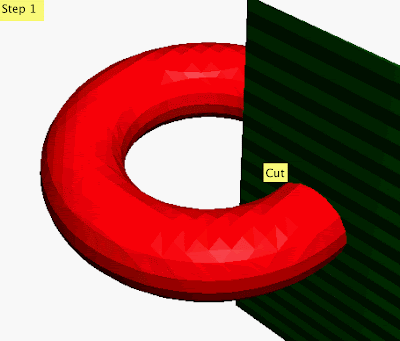In the first step, the cut goes through the torus and creates two ends. The resulting figure can be straightened out into a cylinder.A second cut is made parallel to the surface to the cylinder along the plane indicated. The cylinder can then be unrolled into a rectangle. The result of this process is summarized in the following diagram.The fundamental polygon for the torus. It has four sides, and can therefore be stretched into a square continuously, as it is shown here. The orientation and lettering of the sides indicates which way the boundaries of the square are connected to produce the manifold. The sides with the same letter are connected to each other so in such a way that the arrows face the same direction. Here the top and bottom are connected, and then the left and right. It is easy to see that this is the reverse of the process that we used to decompose the torus.

The same process can be applied to the sphere. The fundamental polygon for the sphere is again a square, but it is connected in a rather different way.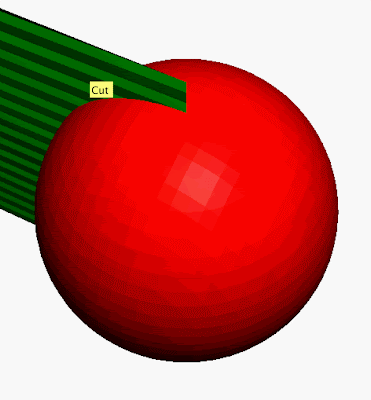The above cut is made before "flattening" the sphere into the plane figure below.This fundamental polygon has the same structure as that of the torus, but is connected differently. The points of each arrow must line up in the end result. With a square piece of paper, one only has to fold diagonally to line up the indicated arrows. The figure is then "inflated" to produce the sphere.

The fundamental polygons can be written as follows. Going in a clockwise direction from the upper left corner, the fundamental polygon for the torus is written ABA*B* and, for the sphere, ABB*A* (the * in each case represents an arrow pointing in the counterclockwise direction).

Next, it is possible to extend this system to other two-manifolds, and even to other dimensions. A closer examination of these figures gives insight into curvature as well.

Sources:
http://whites-geometry-wiki.wikispaces.com, http://svr225.stepx.com:3388/sphere, http://en.wikipedia.org/wiki/Fundamental_polygon